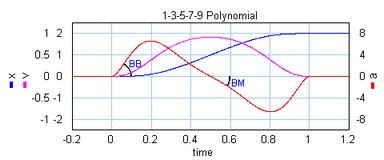﻿ 20-sim webhelp > Toolboxes > Mechatronics Toolbox > Cam Wizard > Cam Motion Profiles

# Cam Motion Profiles

## Motion Types

Two types of motion profiles can be generated by the Cam wizard: intermittent steps and continuous pulses. The intermittent step motion does not return to its starting position, but gradually moves further away . It is characterized by the parameters stroke, start_angle and stop_angle.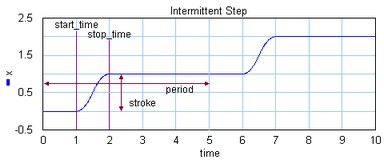The continuous pulse motion output returns at the end of each cycle returns to the starting position. It is characterized by the parameters stroke, start_angle, stop_angle, return_angle and end_angle.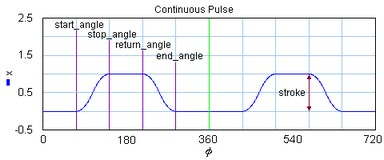## Motion Profiles

In mechanical engineering an important part of design is the reduction of vibrations. Every time a construction is in motion, vibrations are induced. When cams or mechanisms are used, the amount of vibrations, depend on the kind of motion that is induced. E.g. a sudden step change will induce violent vibrations in comparison with with a fluent motion. An important parameter to characterize motions, is the order:

 1 Zero Order: Motions that are discontinuous in the position (e.g. a step).
 2 First Order: Motions that are continuous in the position but discontinuous in the velocity (e.g. a ramp).
 3 Second Order: Motions that are continuous in the position and velocity but discontinuous in the acceleration.
 4 Third Order: Motions that are continuous in the position, velocity and acceleration.
 5 Fourth Order: Motions that are continuous in the position, velocity, acceleration and jerk.

The specific shape of a motion profile can have a significant influence on the dynamic behavior. Some profiles minimize the maximum velocity, some profile minimize acceleration, while other profiles tend to make a tradeoff between the maximum velocity and acceleration. If we take a standard motion with stroke 1 and motion time 1 sec., the following table can be generated:

 profile order vmax amax y(0) y(1/2) Ramp 1 1 infinite infinite 0 2 > 1.57 > 4.93 infinite < -15.5 Trapezoidal 2 2 4 infinite -infinite 2 1.67 4.17 infinite 0 Geneva Mechanism 2 2.41 8.49 infinite -118.5 Sine 2 1.57 4.93 infinite -15.5 Cubic 3 2 4 32 -32 3 1.67 5.55 55.6 0 Cycloidal 3 2 6.28 39.5 -39.5 Modified Sine 3 1.76 5.53 69.4 -23.2 Modified Trapezoidal 3 2 4.88 61.4 -61.4 MSC50 3 1.26 9.20 173.6 0 MSC%4 3 1.37 7.20 113.1 0 3-4-5 Polynomial 3 1.88 5.77 60 -30 3/4 2.05 10.25 BB BM

1: Parameter CV = 20%

2: For lw >> lc the Crank Rod profile equals the sine profile, for lw > lc performance deteriorates.

3: Parameter CV = 20%, CA = 20%

4: Parameter n = 30%, alpha = 10%

5: Parameter BB = 30%, BM = 10%

Here y(0) is the initial jerk (derivative of acceleration) and y(1/2) the crossover (halftime) jerk.

## Ramp

The ramp profile is a first order profile. It has a constant velocity and acceleration peaks at the start and end of the motion.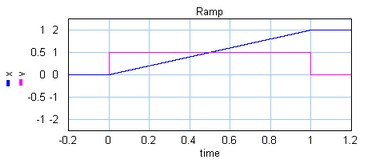Crank Rod Mechanism

A Crank Rod mechanism converts a rotary motion into a repeating translation and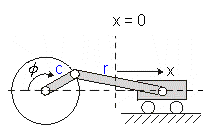If the rod is much longer than the crank, the resulting motion profile will approach the sine profile. Below the motion profile is shown for half a rotation of the crank (angle from 0 to 180 degrees in 1 sec.).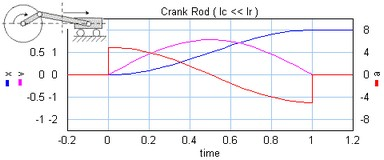If the rod gets smaller, the maximum velocity and acceleration increase.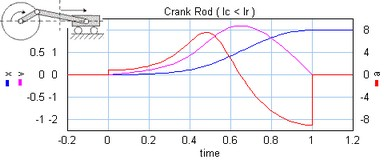Note: A real crank-rod mechanism will not suddenly stop at half a rotation and therefore not show a discontinuous acceleration.

## Trapezoidal

The trapezoidal profile is a second order profile. It has a constant acceleration at the start of the motion and a constant deceleration at the end of the motion.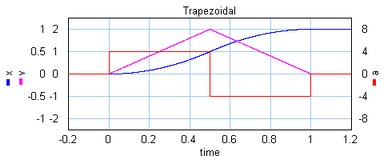This profile is most widely used in early servo systems. Because of the discontinuity in the acceleration this motion profile can still induce a lot of vibrations. Therefore in modern servo systems third order profiles are preferred.

## Partial Trapezoidal

The partial trapezoidal profile is a second order profile. It has is equal to the trapezoidal motion, but has a constant velocity part, during a fraction CV (%) of the motion.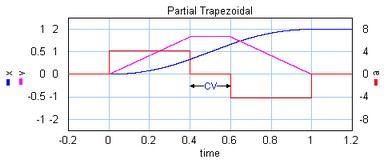## Geneva Mechanism

A Geneva mechanism is an old motion profile generation mechanism. A continuously rotating crankshaft (a) generates an intermittent motion of a second shaft (b). The result is a second order profile.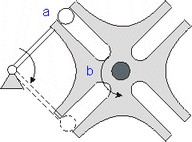The motion profile generator yields the motion of the second shaft (b). It is shown below: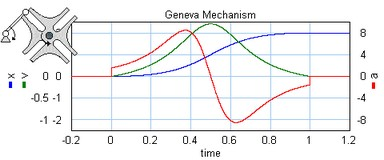## Sine

The sine profile is a second order profile of which the displacement can be described as:

x = 0.5 - cos (p * t) / 2.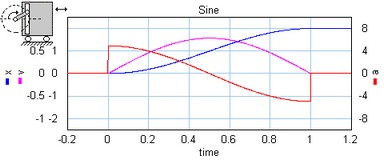## Cubic

The cubic profile is a third order profile of which the acceleration is constantly increasing and decreasing.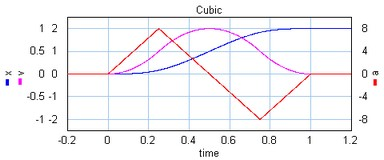## Partial Cubic

The partial cubic profile (3rd order) is a modified cubic profile with a constant velocity during a fraction CV (%) of the motion and a constant acceleration during a fraction CA (%) of the motion.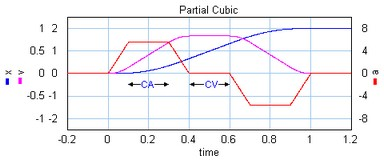## Cycloidal

The cycloidal profile is a third order profile, of which the velocity can be described as:

v = stroke*(1-cos(t*a))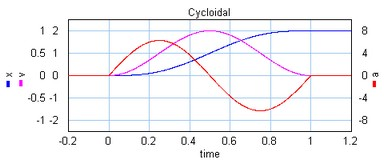## Modified Sine

The modified sine profile is also a third order profile. It is a modification of the cycloidal profile to get a lower maximum velocity and a lower maximum acceleration.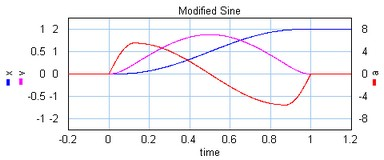## Modified Trapezoidal

The modified trapezoidal profile is a modification of the trapezoidal profile (to make it a third order profile). This profile yields a very low maximum acceleration.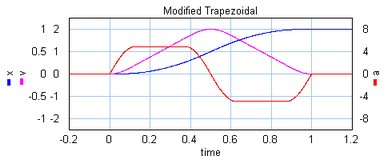## Modified Sine with Constant Velocity (MSC50)

The modified sine with constant velocity profile (3rd order) is a modification of the modified sine profile. It has a constant velocity during 50% of the motion.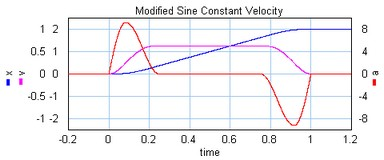## General Modified Sine with Constant Velocity (MSC%)

The modified sine with constant velocity profile (3rd order) is a modification of the modified sine profile. It has a constant velocity during a user definable part of the of the motion. The non-zero acceleration part is defined by two parameters which are both defined as a fraction of the motion. The first parameters alpha (%) defines the start of the acceleration and the second parameters n (%) defines the end of the acceleration.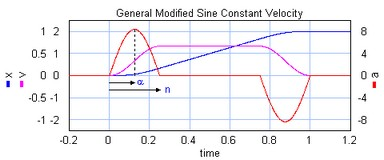## 3-4-5 Polynomial

The is a third profile described by a 5th order polynomial.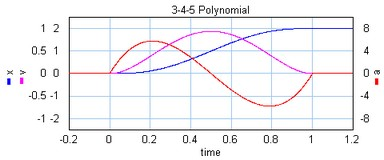## 1-3-5-7-9 Polynomial

The is a third/fourth order profile described by a 9th order polynomial. The profile is characterized by two parameters that denote the initial jerk, BB, and the crossover jerk, BM. If BB is chosen zero, this a a fourth order profile. If BB is chosen non-zero, this is a third order profile.